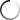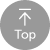B1 中級 6

 So in this video, I want to show you an example of exactly how information is encoded on a real network link. So this is a setup that I put together, have my computer here connected to an Ethernet network and have stripped away the Ethernet cable like this. So if we look inside the cable, we can see there's like four pairs of wires. There's an orange pair of blue pair of brown pair and a green pair. And now, in this set up, the other end of this cable is connected to a 10 based T and bass T network, which is a particular standard that's developed by the IEEE. The I Triple E is the Institute for Electrical and Electronics Engineers and ah, 10 based. He is. It is kind of an older standard that allows you to transmit data at 10 megabits per 2nd 10 megabits per second, which is how we write this, which is 10 million bits per second, which may sound like a lot, but the same even it poured on. My computer also supports a couple other standards, reports 100 face Lips 100 based T X, which allows you to send data at 100 megabits per second, and it also supports 1000 based T, which lets you transmit data at at one gigabit per second or one billion bits per second. But for this experiment, I'm gonna use 10 based T because it's a relatively basic uses a belt relatively basic line coping mechanism, which is Manchester encoding, which we which we saw in the last video. These other two standards are a bit more complex. So for this video for this demonstration, I just want to use this this sort of relatively simpler standard. So of these of these three standards, I guess the 1000 base T the one gigabit or gigabit Ethernet standard, is the only one that actually uses all four pairs of wires in here. The other two standards only used two pairs. So from the computers perspective, the orange pair in this case is used for transmitting data in this direction. And the green pear is used for receiving data, and then the blue and the and the brown pairs are unused. So what I've done here is stripped away a little bit of the insulation on the orange pear and ah from the I guess the two different wires here in the orange pear and connected in a telescope, which is this thing here, have connected this a silla scope to to the to the orange pair to see exactly what's going on. And what the telescope does is it measures the voltage across the two wires in the orange pears, and it plots that voltage overtime on this graph. Um, and so here we can see, the voltage seems to be going up and down a lot over time. So if I scroll down here, what I've done has taken a screenshot from the oscilloscope so we can look at this in a bit more detail. Okay, so to orient you with what's going on here, This center line here across the middle is zero volts. And this key here is telling us that each division is 500 mil if ALTs. So this is 500 million volts. This is 500 million volts per division, which means two divisions is going to be one volt. Um, and then down here, this is gonna be negative one volt. And so what we can see is that the signal is fluctuating between positive one volt and negative one volt positive, one volt native involved and so on Now in the horizontal direction. This is telling us that each division represents 100 nanoseconds. So from here to here, for example, is 100 Nana seconds. And just to remind ourselves, one nanosecond is equal to 10 to the minus nine seconds. Um and if we I guess we could multiply both sides of this by 100 to see that 100 Nana seconds is equal to 10 to the minus seven seconds. Or another way to look at this is to say that 100 Nina seconds is equal to one over 10 to the seven seconds. Or you could also write that is one over, uh, 10 million seconds. We're 1 10 millionth of a second there. Another way to think of this is that basically, each of these divisions is 1 10 millionth of a second. Or, in other words, you're gonna have 10 million of these divisions per second. Remember, the speed of this Ethernet link is 10 megabits per second, and so 10 megabits per second is 10 million bits per second. And so we're basically going to expect to see 10,000,001 bit every 100 nanoseconds. So this is a very convenient division here. We should be able to find one bit per per division. Um and so what's going on here is we're using the same Manchester and coating that we saw the last time, and so the signal looks a little bit different. But really, what's going on is we have the same symbols where the symbol for a zero is a voltage transition from a positive voltage to a negative voltage. And then the symbol for one is a transition from a negative voltage to a positive voltage. And so these symbols are happening at this at the centre time here, where that bolted transition is taking place. And so if we want to, we can just start looking at the at the signal and see if we can see some of these patterns here. So right here, for example, we see a voltage transitioning from a negative voltage to a positive all to try here on, so that would indicate that that is a one. And then if we go another 190 seconds, we see that same transition from a negative voltage to a positive voltage. And that means that's another one going from negative to positive. And so here in the middle, we have this transition going back down from positive to negative. But because that's not happening 100 Nana seconds apart. We know to ignore that because we know that the link speed is 100 megabits per second, which means we should expect a symbol per 100 nanoseconds. So even if our clock is a little bit off, we should be able to tell the difference between 59 seconds 890 seconds. And so, if we keep going now, we get to a transition where we're going from a positive voltage to a negative voltage and that's a zero. And here we're going from negative to positive. So that's the one. Positive to Negative is a zero positive to negative again is a zero negative. To positive is a one, and positive to negative is a zero. And so here we've we've we've decoded eight bits and eight bits, makes a bite, and so we've decoded a bite of information from the Internet. But if we wantto want to, we can keep going. Um, I've taken another screen shot and just kind of upended it here and lined it up. So it all lines up perfectly. And so if we keep going, we see that we get another, uh, Looks like a one, actually. Right here. Followed by a zero. Another zero. Another zero. Another zero. We're going Positives, Negatives that zero Another positive to negative, which is a zero. And then a negative to positive. This is a one and then a positive to negative, which is a zero. And so this is another eight bits or another bite, so we could keep going. But we've decoded two bites here from from this Ethernet. And one thing that sort of, I guess a little bit different about Ethernet. Is that the way that it puts thes bits onto the wire? In this format, it reverses the order of the bits. So in other words, the sort of the 120 eight's place is sent This sent last and the ones place has sent first. So if we want to convert this to a real binary number, we actually have to flip all these bits around. So the zero goes first. Then the one than this zero than this zero than this one than this zero. And then the one and the ones we've just flipped thes bits from first to last and then the same thing for for this. So we start over here and just go backward. Zero 10000 That's five zeros. And then finally this one. And if we look at these two, these two bites here and convert these two decimal, this one, if we convert to decimal, we get 75 and this one we get a 65. And this is actually the information that I was trying to send on my Ethernet interface that I captured here. Um, and actually, if you were to use that asking table that we looked at a couple videos ago. 75 is stands for the letter K and 65 stands for the letter A. And this is exactly the date I was trying to send.

# Analyzing actual Ethernet encoding | Networking tutorial (4 of 13)

6

#### 影片學習單字重點#### 影片討論1. ## 1. 單字查詢

在字幕上選取單字即可即時查詢單字喔！

2. ## 2. 單句重複播放

可重複聽取一句單句，加強聽力！

3. ## 3. 使用快速鍵

使用影片快速鍵，讓學習更有效率！

4. ## 4. 關閉語言字幕

進階版練習可關閉字幕純聽英文哦！

5. ## 5. 內嵌播放器

可以將英文字幕學習播放器內嵌到部落格等地方喔

6. ## 6. 展開播放器

可隱藏右方全文及字典欄位，觀看影片更舒適！

1. ## 英文聽力測驗

挑戰字幕英文聽力測驗！

1. 點擊展開筆記本讓你看的更舒服

1. UrbanDictionary 俚語字典整合查詢。一般字典查詢不到你滿意的解譯，不妨使用「俚語字典」，或許會讓你有滿意的答案喔